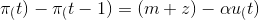### Create an Account

Already have account?

### Forgot Your Password ?

Home / Questions / If the Phillips curve equation is represented by equation: \pi _(t)-\pi _(t-1)=(m+z)-\alph...

# If the Phillips curve equation is represented by equation: \pi _(t)-\pi _(t-1)=(m+z)-\alpha u_(t) , with the change in the inflation rate equal to m plus z minus the product of alpha and u, which of t

If the Phillips curve equation is represented by equation:, with the change in the inflation rate equal to m plus z minus the product of alpha and u, which of the following will cause a reduction in the natural rate of unemployment?

a. an increase in m

b. an increase in z

c. an increase in alpha

d. an increase in actual inflation

e. an increase in expected inflation

Apr 16 2021 View more View Less

#### Answer (Solved)Subscribe To Get Solution